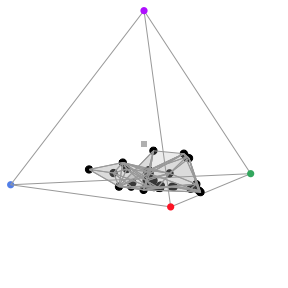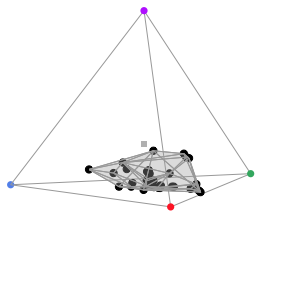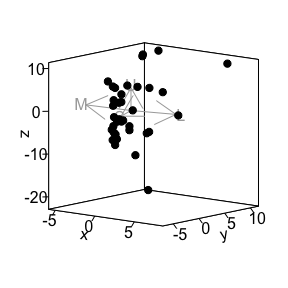# Colour volume estimation

#### 2020-11-12

Traditionally, colour volume or “colourfulness” of an object has been measured by computing the volume of the convex hull containing the data points, as described in (Stoddard and Prum 2008)

$$\alpha$$-shapes are a new tool available in pavo to estimate colour volumes, while allowing the presence of voids and pockets, which may lead to a more accurate measurement of “colourfulness” than convex hulls. For more information on the theoretical background, please report to the related article (Gruson n.d.)

# $$\alpha$$-shapes in chromaticity diagrams

You can plot the colour volume using $$\alpha$$-shape with the vol() function (for non-interactive plots, tcsvol() otherwise) by specifying type = "alpha". By default, this will use the $$\alpha^*$$ value defined in Gruson (n.d.).

library(pavo)
data(flowers)
vis_flowers <- vismodel(flowers, visual = "avg.uv")
tcs_flowers <- colspace(vis_flowers)
plot(tcs_flowers)
vol(tcs_flowers, type = "alpha")
#> 'avalue' automatically set to 1.8275e-01To get the colour volume value, you can use the summary.colspace() function. The colour volume computed by the convex hull is contained in the c.vol column and the colour volume computed by the $$\alpha$$-shape (with parameter $$\alpha^*$$) is contained in the a.vol column:

summary(tcs_flowers)
#> Colorspace & visual model options:
#>  * Colorspace: tcs
#>  * Quantal catch: Qi
#>  * Visual system, chromatic: avg.uv
#>  * Visual system, achromatic: none
#>  * Illuminant: ideal, scale = 1 (von Kries colour correction not applied)
#>  * Background: ideal
#>  * Relative: TRUE
#>  * Max possible chromatic volume: 0.215735
#> 'avalue' automatically set to 1.8275e-01
#>            centroid.u centroid.s centroid.m centroid.l      c.vol  rel.c.vol
#> all.points 0.06025649  0.2375617   0.317973  0.3842088 0.01432726 0.06617479
#>            colspan.m  colspan.v huedisp.m huedisp.v   mean.ra   max.ra
#> all.points 0.2000963 0.01309332 0.7414951 0.2235526 0.8319508 0.999151
#>                  a.vol
#> all.points 0.006057976

Alternatively, you can set the $$\alpha$$ parameter to the value of your choice via the avalue argument:

plot(tcs_flowers)
vol(tcs_flowers, type = "alpha", avalue = 0.5)# $$\alpha$$-shapes in perceptually uniform spaces

In the previous section, we focused on $$\alpha$$-shapes in chromaticity diagrams since it is the most common space where convex hulls (that $$\alpha$$-shapes aim at replacing) are used. But it is also possible to use $$\alpha$$-shapes in other spaces, such as perceptually uniform spaces.

Let’s first build this uniform space and look at the data points in this space:

cd_flowers <- coldist(vis_flowers)
#> Warning: Quantum catch are relative, distances may not be meaningful
#> Calculating noise-weighted Euclidean distances
xy_flowers <- jnd2xyz(cd_flowers)
plot(xy_flowers)High-level functions to build the $$\alpha$$-shape directly in pavo have not yet been implemented but you can use the alphashape3d package directly to compute the $$\alpha$$-shapes, its volume and display it in a 3D interactive plot.

library(alphashape3d)
ashape_jnd <- ashape3d(as.matrix(xy_flowers), alpha = 10)
volume_ashape3d(ashape_jnd)
#>  748.863
plot(ashape_jnd)
#> Device  1  : alpha =  10
#> Warning in rgl.material(...): partial argument match of 'col' to 'color'

#> Warning in rgl.material(...): partial argument match of 'col' to 'color'

#> Warning in rgl.material(...): partial argument match of 'col' to 'color'

# Colour volume overlap with $$\alpha$$-shapes

$$\alpha$$-shapes can also be used to measure the colour similarity of two objects, by computing the colour volume overlap. This is done in pavo with the overlap3d() function. For example, let’s compare the colour volume of the crown and the breast of stripe-tailed yellow finches (Sicalis citrina):

data(sicalis)
tcs.sicalis.C <- subset(colspace(vismodel(sicalis)), "C")
tcs.sicalis.B <- subset(colspace(vismodel(sicalis)), "B")
voloverlap(tcs.sicalis.C, tcs.sicalis.B, type = "alpha", plot = TRUE)
#> 'avalue' automatically set to 2.4445e-02
#> 'avalue' automatically set to 2.6255e-01
#> Warning in overlap3d(colsp1, colsp2, avalue, plot, interactive, col, fill, :
#> interactive = FALSE has not been implemented yet, falling back to interactive
#> plot.
#> Warning in f(...): partial argument match of 'col' to 'color'

#> Warning in f(...): partial argument match of 'col' to 'color'
#>           vol1         vol2 s_in1 s_in2 s_inboth s_ineither psmallest pboth
#> 1 1.849436e-06 4.586381e-06     3     8        0         11         0     0